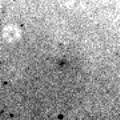# 141P/Machholz 2 (1999)###Profile

 Designation 141P/1999 P1 Recovery Date August 3, 1999 Magnitude 20.8 mag Recoverer R. H. McNaught

###Pictures###Orbital Elements

``` Comet 141P/Machholz 2 ( Nucleus A )

The following improved orbital elements by Kenji Muraoka, are
from 234 observations  1994 to 2000, including 9 Planets, Moon
and 5 minor planets perturbations and non-gravitational effect
of style II.   The mean residual is +/- 0.83 arc seconds.

Epoch  =  1999 Dec.  8.0  TT       JDT = 2451520.5
T  =  1999 Dec.  9.27438       +/- 0.00006 (m.e.) TT
Peri. =  149.30205                +/- 0.00018
Node  =  246.13618                +/- 0.00013   (2000.0)
Incl. =   12.81126                +/- 0.00003
q  =    0.7489322              +/- 0.0000005 AU
e  =    0.7510566              +/- 0.0000006
a  =    3.0084442              +/- 0.0000025 AU
n  =    0.18888225             +/- 0.00000024
P  =    5.218                  +/- 0.0000065  years
A1  =   +0.504                  +/- 0.020
A2  =   -0.19475                +/- 0.00325

Comet 141P/Machholz 2 ( Nucleus D )

The following improved orbital elements by Kenji Muraoka,
are from 114 observations 1994 to 1999, including 9 Planets,
Moon and 5 minor planets perturbations and non-gravitational
effect of style II.  The mean residual is +/- 1.09 arc seconds.

Epoch  =  1999 Dec.  8.0  TT       JDT = 2451520.5
T  =  1999 Dec.  9.95662       +/- 0.00025 (m.e.) TT
Peri. =  149.29339                +/- 0.00088
Node  =  246.13491                +/- 0.00040   (2000.0)
Incl. =   12.81195                +/- 0.00012
q  =    0.7489913              +/- 0.0000037 AU
e  =    0.7510778              +/- 0.0000081
a  =    3.0089376              +/- 0.0000331 AU
n  =    0.18883579             +/- 0.00000311
P  =    5.219                  +/- 0.0000861  years
A1  =   +1.605                  +/- 0.146
A2  =   +0.42056                +/- 0.04229
```

###Finding Charts###Magnitudes Graph

```        m1 = 14 + 5 log d + 20 log r(t - 10)

Component A:
m1 = 14 + 5 log d + 20 log r(t - 10)

Component D:
m1 = 18.5 + 5 log d + 55 log r(t + 20)
```##### The orbital elements are calculated by Kenji Muraoka. The charts are made with StellaNavigator Ver.2.0 for Windows (AstroArts / ASCII). The magnitudes graphs are made with Comet for Windows.